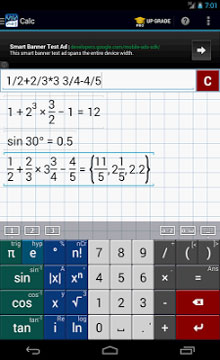# Min Oaker

(ဘီလူးကၽြန္းသားေလး) နည္းပညာ

## Friday, October 17, 2014သခ်ၤာ/ရူပ/ဓာတု/ပထ၀ီ ေက်ာင္းသားေတြလိုအပ္ေနတဲ့ေဆာ့၀ဲေလး Graphing Calculator by Mathlab ေလးပါ
ဗ်ာ ပ့ုံမွာၾကည့္တာနဲံ ဘာေတြပါ၀င္လဲသိမွာပါ အဆင္ေပျပမဲ့ေဆာ့၀ဲေကာင္းေလးပါ (ပုသိမ္အိုင္တီ)
Graphing calculator with algebra. Essential tool for school and college. Replaces bulky and expensive handheld graphing calculators.
Multiple functions on a graph, polar graphs, graphing of implicit functions, values and slopes, roots, extremes, intersections. Algebra: polynomials, polynomial equation solving, matrices, fractions, derivatives, complex numbers and more. Shows results as you type. Use menu to switch between modes.
Help site with instructions and examples: help.mathlab.us
If you have a question, send email to calc@mathlab.us
SCIENTIFIC CALCULATOR
* arithmetic expressions +,-,*,/,
* square root, cube and higher roots (hold root key)
* exponent, logarithms (ln, log)
* trigonometric functions sin p/2, cos 30, …
* hyperbolic functions sinh, cosh, tanh, … (hold “e” key to switch)
* inverse functions (hold direct function key)
* complex numbers, all functions support complex arguments
* derivatives sin x’ = cos x, … (hold x^n key)
* scientific notation (enable in menu)
* percent mode
GRAPHING CALCULATOR
* multiple functions graphing
* implicit functions up to 2nd degree (ellipse 2x^2+3y^2=1, etc.)
* polar graphs (r=cos2?)
* parametric functions, enter each on new line (x=cos t, y=sin t)
* function roots and critical points on a graph, tap legend to turn on and off (top left corner), use menu to display as a list
* graph intersections (x^2=x+1)
* tracing function values and slopes
* scrollable and resizable graphs
* pinch to zoom
* fullscreen graphs in landscape orientation
* function tables
* save graphs as images
* save tables as csv
FRACTION CALCULATOR
* simple and complex fractions 1/2 + 1/3 = 5/6
* mixed numbers, use space to enter values 3 1/2
ALGEBRA CALCULATOR
* linear equations x+1=2 -> x=1
* quadratic equations x^2-1=0 -> x=-1,1
* approximate roots of higher polynomials
* systems of linear equations, write one equation per line, x1+x2=1, x1-x2=2
* polynomial long division
* polynomial expansion, factoring
MATRIX CALCULATOR
* matrix and vector operations
* dot product (hold *), cross product
* determinant, inverse, norm, transpose, trace
LIBRARY
* user defined constants and functions (PRO)